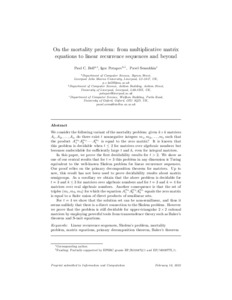# On the Mortality Problem: From Multiplicative Matrix Equations to Linear Recurrence Sequences and Beyond

Bell, PC, Potapov, I and Semukhin, P (2021) On the Mortality Problem: From Multiplicative Matrix Equations to Linear Recurrence Sequences and Beyond. Information and Computation. ISSN 0890-5401Preview
Text
BPSInC21.pdf - Accepted Version
We consider a variant of the mortality problem: given matrices $A_1, ..., A_t$, do there exist nonnegative integers $m_1, \ldots, m_t$ such that $A_1^{m_1} \cdots A_t^{m_t}$ equals the zero matrix? This problem is known to be decidable when $t \leq 2$ but undecidable for integer matrices with sufficiently large $t$ and $k$. We prove that for t=3 this problem is Turing-equivalent to Skolem's problem and thus decidable for k <= 3 (resp. k=4) over (resp. real) algebraic numbers. Consequently, the set of triples $(m_1, m_2, m_3)$ for which the equation $A_1^{m_1}A_2^{m_2}A_3^{m_3}$ equals the zero matrix is a finite union of direct products of semilinear sets. For t=4 we show that the solution set can be non-semilinear, and thus there is unlikely to be a connection to Skolem's problem. We prove decidability for upper-triangular 2x2 rational matrices by employing powerful tools from transcendence theory such as Baker's theorem and S-unit equations.View Item